Problem 2: Unequal errors

In the foregoing discussion, I assumed that all of the individual yi values had precisely the same typical expected error, quantified by the so-called "standard error," which is defined as the Gaussianin the probability distribution for. (Note that the standard error is sometimes referred to as the "mean error" - standard error and mean error mean the same thing. They are not the same thing as the "probable error," which you will sometimes see mentioned in older books and papers. The probable error is defined as the half-length of a 50% confidence interval: when you give a numerical value for your estimate of some physical quantity and quote a probable error, you are saying that you think there is a 50% chance that the true value of that quantity is within the stated error bars. The standard error = the mean error is the half-length of a 68.3% confidence interval: when you quote a standard or mean error, you are saying that you think there's slightly over a two-thirds chance that the true value is contained within your error bars. This latter is the current standard astronomical convention.)

In real life, it is commonly the case that the individual observations have different known or estimated standard errors,i. This situation is nearly as easy to deal with as the case of equal errors. We now write the Gaussian function as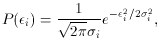so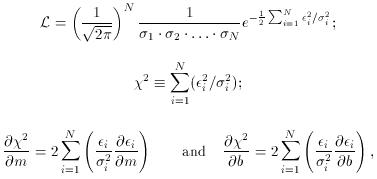where I have been very careful to keep the individual's throughout. Let us define the weight of an observation as wi= 2 s2 /i2, where s2 is just some arbitrary constant that you can pull out of a hat; I have included it for generality and I have written it as s2 to emphasize that it should be a positive constant. And furthermore, . . . well, wait just a bit. Our conditions for a minimum of2 are:You can see now that the specific value that you adopt for the arbitrary constant s2 doesn't matter at all - since the summations are going to be set equal to zero anyway, whatever value of s2 you use, it can be pulled out of the summations and the equations are still true. In matrix form we now haveor, in algebraic form,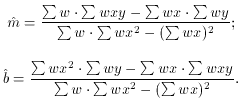In this case,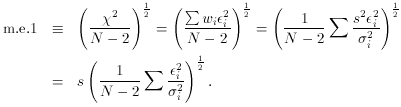If you have used correct values for all thei2, then (1 / (N - 2)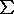i2 /i2) is a so-called "chi-squared" variable with an expected value of unity; it will equal unity more and more precisely for larger and larger sample sizes, N. Thus, if thei are correct, after you have performed your least-squares fit you should wind up with m.e.1s. Recalling that s is by definition theof an observation of weight 1 (since ws2 /2), we can now see why m.e.1 is called the "mean error of unit weight": it is the mean error, or the correct value of, corresponding to a data point with w = 1. If we are uncertain whether our assumed values of thei are correct, we can use the derived m.e.1 as a guide. We start off by setting s1. Then, if our values ofare correct, the derived m.e.1 should come out to have a value near 1.0. If, on the other hand, the m.e.1 comes out with a value near 2.0, we would suspect that we have underestimated our errors by a factor of two. On the other hand, in many cases we do not know the true errors of all our observations, but we have a good handle on their relative errors: we may know that observation number 2 has atwice as large as observation number 1, while not knowing what1 and2 are, really. In this case, we can arbitrarily assign observation 1 unit weight, and observation 2 weight 1/4 (since weight-2. In this case m.e.1 will not come out to unity, it will come out to an estimate of what1 ("the mean error of an observation of unit weight") actually should have been.